# 978-0073382395 Chapter 8 Questions and Problems 11-22

Document Type
Homework Help
Book Title
Fundamentals of Corporate Finance Standard Edition 9th Edition
Authors
Stephen Ross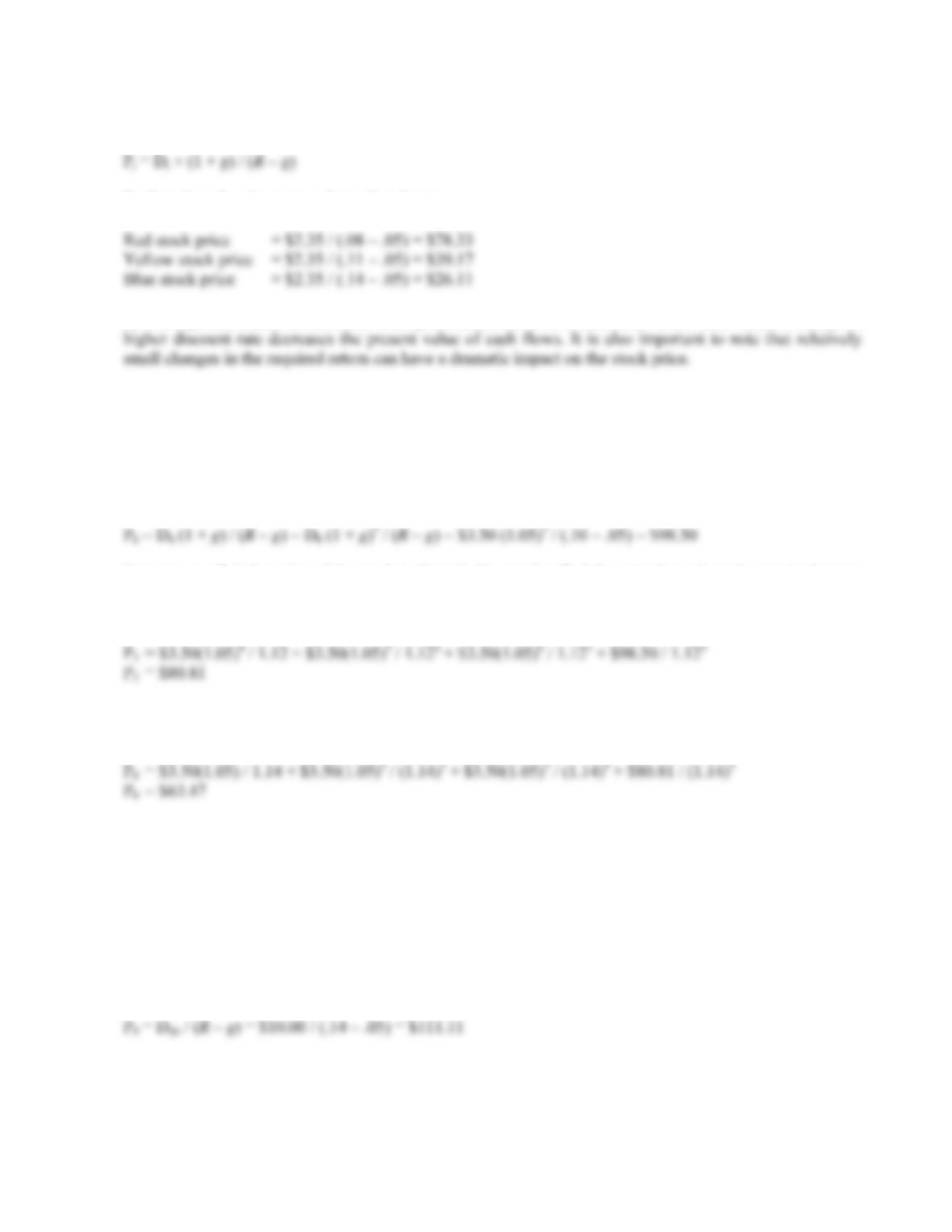B-144 SOLUTIONS
9. We can use the constant dividend growth model, which is:
P
So the price of each company’s stock today is:
As the required return increases, the stock price decreases. This is a function of the time value of money: A
Intermediate
10. This stock has a constant growth rate of dividends, but the required return changes twice. To find the value
of the stock today, we will begin by finding the price of the stock at Year 6, when both the dividend growth
rate and the required return are stable forever. The price of the stock in Year 6 will be the dividend in Year
7, divided by the required return minus the growth rate in dividends. So:
P
Now we can find the price of the stock in Year 3. We need to find the price here since the required return
changes at that time. The price of the stock in Year 3 is the PV of the dividends in Years 4, 5, and 6, plus
the PV of the stock price in Year 6. The price of the stock in Year 3 is:
P
Finally, we can find the price of the stock today. The price today will be the PV of the dividends in Years
1, 2, and 3, plus the PV of the stock in Year 3. The price of the stock today is:
P
11. Here we have a stock that pays no dividends for 10 years. Once the stock begins paying dividends, it will
have a constant growth rate of dividends. We can use the constant growth model at that point. It is
important to remember that general constant dividend growth formula is:
P
t = [Dt × (1 + g)] / (Rg)
This means that since we will use the dividend in Year 10, we will be finding the stock price in Year 9. The
dividend growth model is similar to the PVA and the PV of a perpetuity: The equation gives you the PV
one period before the first payment. So, the price of the stock in Year 9 will be:
P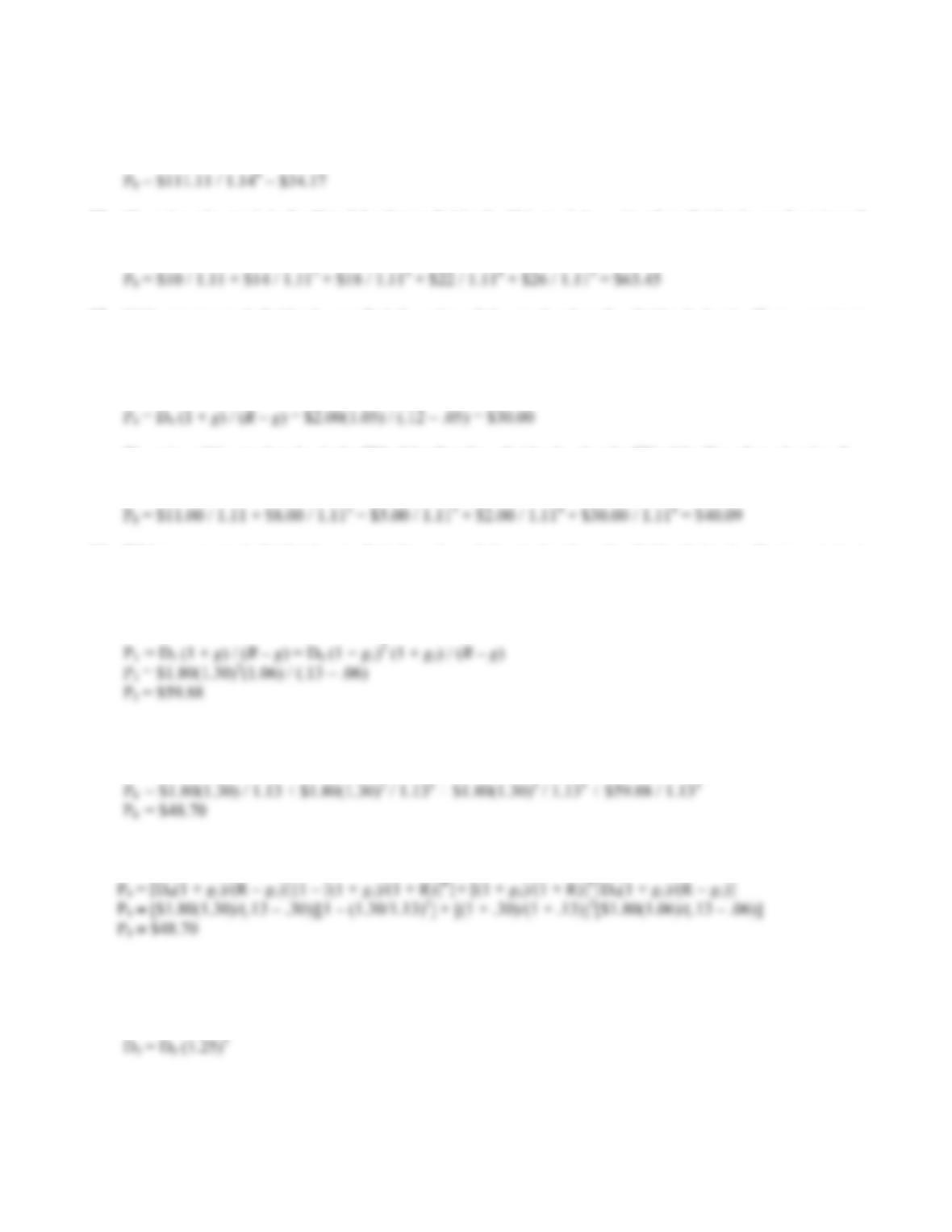CHAPTER 8 B-145
The price of the stock today is simply the PV of the stock price in the future. We simply discount the future
stock price at the required return. The price of the stock today will be:
P
12. The price of a stock is the PV of the future dividends. This stock is paying four dividends, so the price of
the stock is the PV of these dividends using the required return. The price of the stock is:
P
13. With supernormal dividends, we find the price of the stock when the dividends level off at a constant
growth rate, and then find the PV of the future stock price, plus the PV of all dividends during the
supernormal growth period. The stock begins constant growth in Year 4, so we can find the price of the
stock in Year 4, at the beginning of the constant dividend growth, as:
P
The price of the stock today is the PV of the first four dividends, plus the PV of the Year 3 stock price. So,
the price of the stock today will be:
P
14. With supernormal dividends, we find the price of the stock when the dividends level off at a constant
growth rate, and then find the PV of the futures stock price, plus the PV of all dividends during the
supernormal growth period. The stock begins constant growth in Year 4, so we can find the price of the
stock in Year 3, one year before the constant dividend growth begins as:
The price of the stock today is the PV of the first three dividends, plus the PV of the Year 3 stock price.
The price of the stock today will be:
P
We could also use the two-stage dividend growth model for this problem, which is:
P
15. Here we need to find the dividend next year for a stock experiencing supernormal growth. We know the
stock price, the dividend growth rates, and the required return, but not the dividend. First, we need to
realize that the dividend in Year 3 is the current dividend times the FVIF. The dividend in Year 3 will be:
D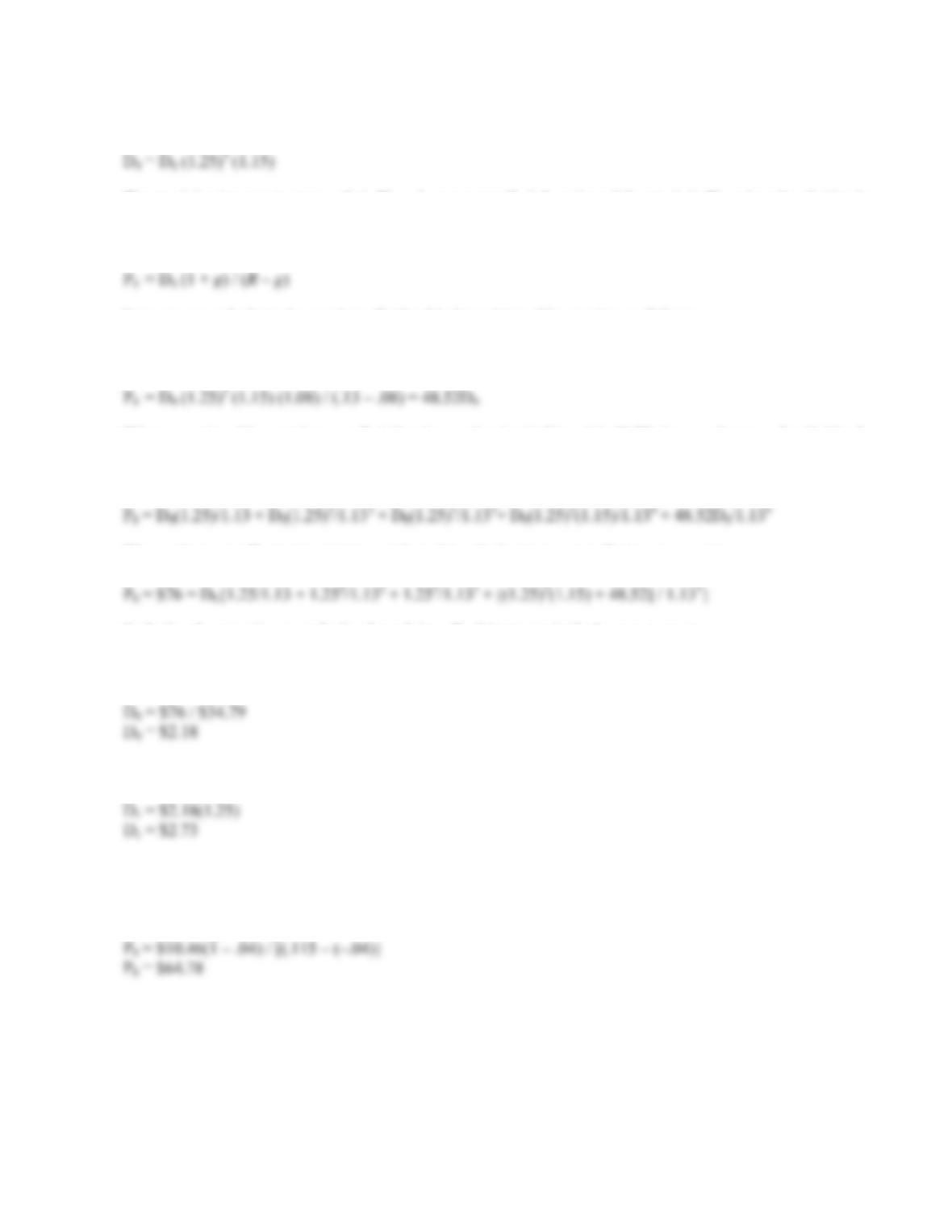B-146 SOLUTIONS
And the dividend in Year 4 will be the dividend in Year 3 times one plus the growth rate, or:
D
The stock begins constant growth in Year 4, so we can find the price of the stock in Year 4 as the dividend
in Year 5, divided by the required return minus the growth rate. The equation for the price of the stock in
Year 4 is:
P
Now we can substitute the previous dividend in Year 4 into this equation as follows:
P
4 = D0 (1 + g1)3 (1 + g2) (1 + g3) / (Rg)
P
When we solve this equation, we find that the stock price in Year 4 is 48.52 times as large as the dividend
today. Now we need to find the equation for the stock price today. The stock price today is the PV of the
dividends in Years 1, 2, 3, and 4, plus the PV of the Year 4 price. So:
P
We can factor out D0 in the equation, and combine the last two terms. Doing so, we get:
P
Reducing the equation even further by solving all of the terms in the braces, we get:
\$76 = \$34.79D0
D
This is the dividend today, so the projected dividend for the next year will be:
D
16. The constant growth model can be applied even if the dividends are declining by a constant percentage, just
make sure to recognize the negative growth. So, the price of the stock today will be:
P
0 = D0 (1 + g) / (R g)
P
17. We are given the stock price, the dividend growth rate, and the required return, and are asked to find the
dividend. Using the constant dividend growth model, we get:
P
0 = \$64 = D0 (1 + g) / (Rg)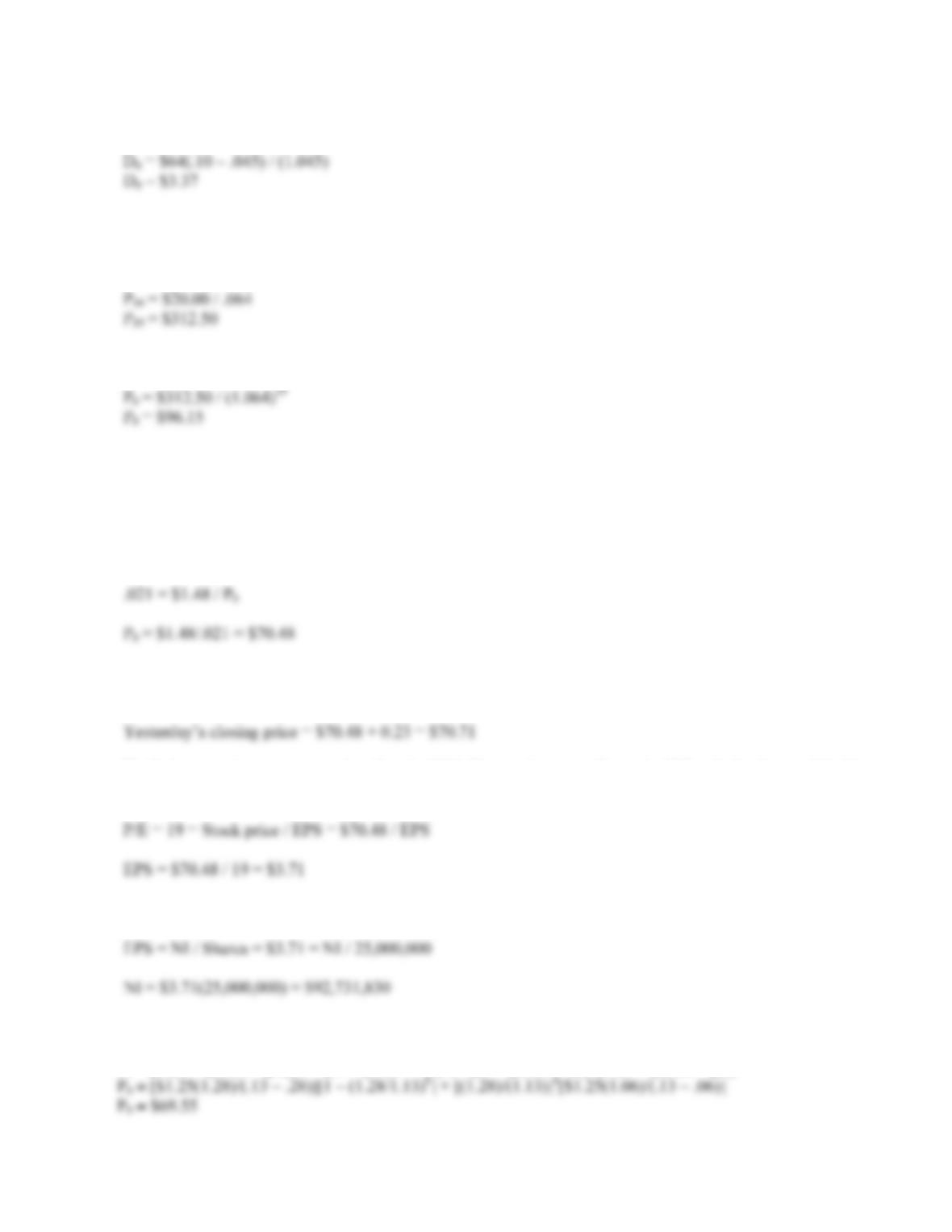CHAPTER 8 B-147
Solving this equation for the dividend gives us:
D
18. The price of a share of preferred stock is the dividend payment divided by the required return. We know the
dividend payment in Year 20, so we can find the price of the stock in Year 19, one year before the first
dividend payment. Doing so, we get:
P
The price of the stock today is the PV of the stock price in the future, so the price today will be:
P
19. The annual dividend paid to stockholders is \$1.48, and the dividend yield is 2.1 percent. Using the equation
for the dividend yield:
Dividend yield = Dividend / Stock price
We can plug the numbers in and solve for the stock price:
The “Net Chg” of the stock shows the stock decreased by \$0.23 on this day, so the closing stock price
yesterday was:
To find the net income, we need to find the EPS. The stock quote tells us the P/E ratio for the stock is 19.
Since we know the stock price as well, we can use the P/E ratio to solve for EPS as follows:
We know that EPS is just the total net income divided by the number of shares outstanding, so:
20. We can use the two-stage dividend growth model for this problem, which is:
P
0 = [D0(1 + g1)/(R – g1)]{1 – [(1 + g1)/(1 + R)]T}+ [(1 + g1)/(1 + R)]T[D0(1 + g2)/(R – g2)]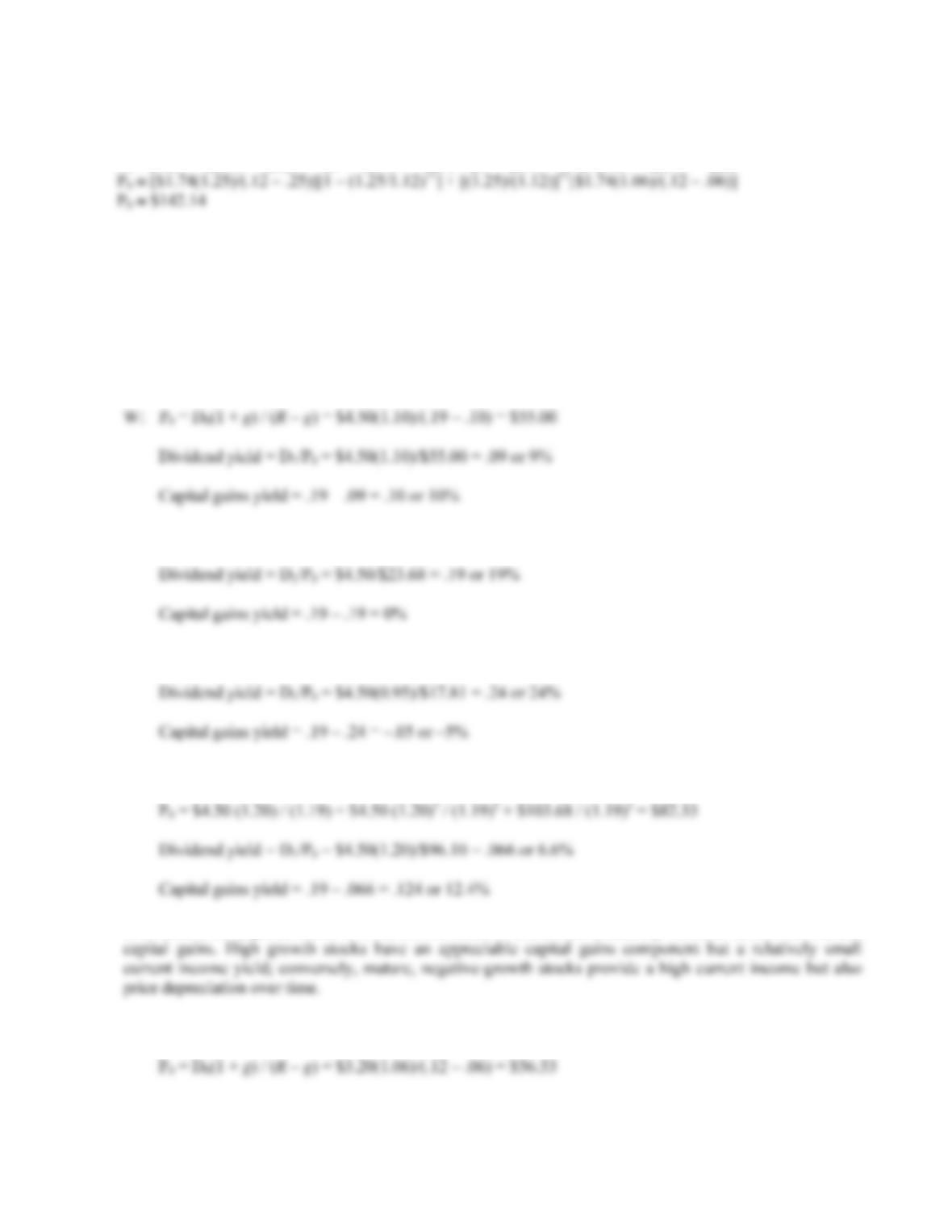B-148 SOLUTIONS
21. We can use the two-stage dividend growth model for this problem, which is:
P
0 = [D0(1 + g1)/(R – g1)]{1 – [(1 + g1)/(1 + R)]T}+ [(1 + g1)/(1 + R)]T[D0(1 + g2)/(R – g2)]
Challenge
22. We are asked to find the dividend yield and capital gains yield for each of the stocks. All of the stocks have
a 15 percent required return, which is the sum of the dividend yield and the capital gains yield. To find the
components of the total return, we need to find the stock price for each stock. Using this stock price and the
dividend, we can calculate the dividend yield. The capital gains yield for the stock will be the total return
(required return) minus the dividend yield.
X: P0 = D0(1 + g) / (Rg) = \$4.50/(.19 – 0) = \$23.68
Y: P0 = D0(1 + g) / (Rg) = \$4.50(1 – .05)/(.19 + .05) = \$17.81
Z: P2 = D2(1 + g) / (Rg) = D0(1 + g1)2(1 + g2)/(Rg2) = \$4.50(1.20)2(1.12)/(.19 – .12) = \$103.68
P
In all cases, the required return is 19%, but the return is distributed differently between current income and
23. a. Using the constant growth model, the price of the stock paying annual dividends will be:
P

## Trusted by Thousands ofStudents

Here are what students say about us.